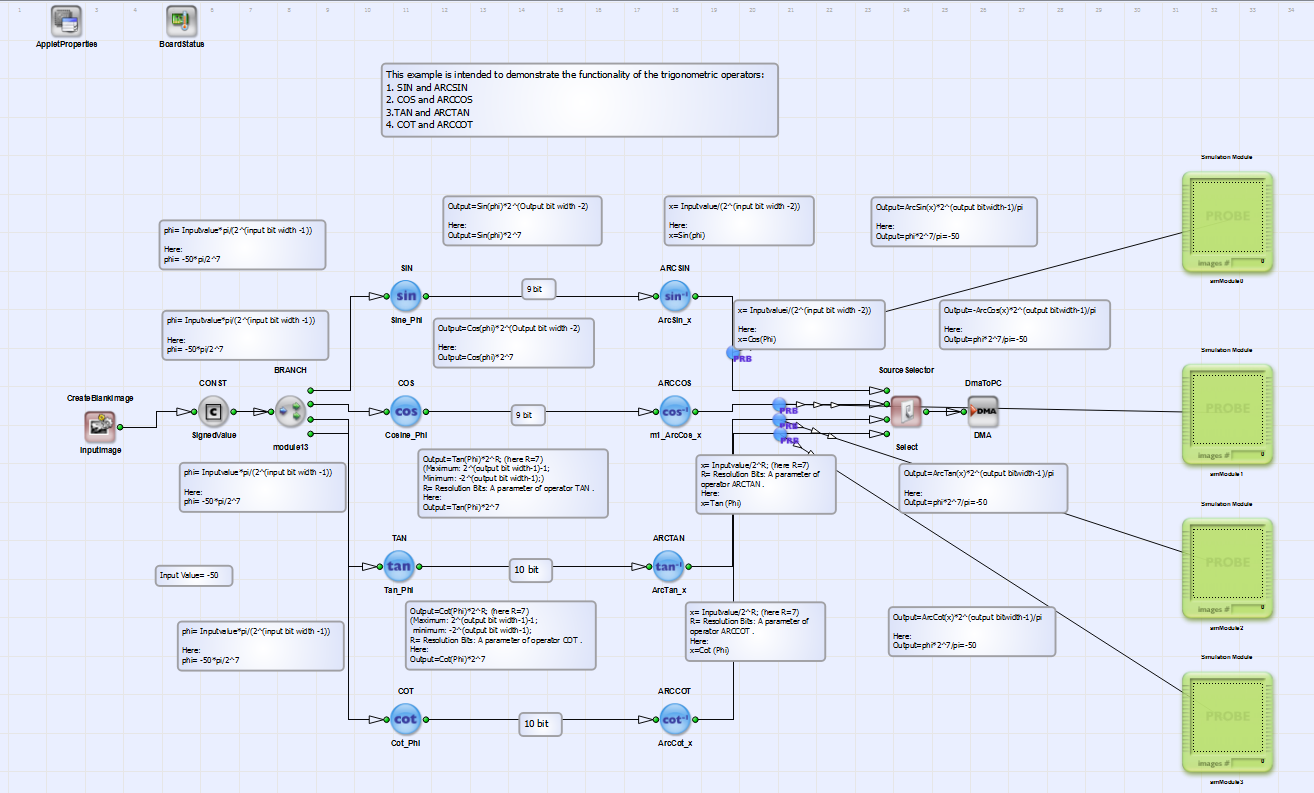## Functional Example for Specific Operators of Library Arithmentics: Trigonometric Functions

Brief Description

Files: examples\OperatorExamples\ArithmeticsLibrary_TrigonometricFunctions.vaDefault Platform: mE5-MA-VCL

Short Description

Demonstration of how to use the operators:

• 1. SIN (library:arithmetics)
• 2. ARCSIN (library:arithmetics)
• 3. COS (library:arithmetics)
• 4. ARCCOS (library:arithmetics)
• 5. TAN (library:arithmetics)
• 6. ARCTAN (library:arithmetics)
• 7. COT
• 8. ARCCOT

This example demonstrates how the specific operators listed above can be used in a functional design. You find a detailed introduction to the arithmetics library in the Operator Reference, section Library Arithmetics.